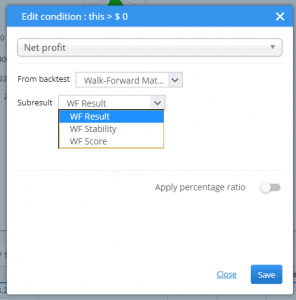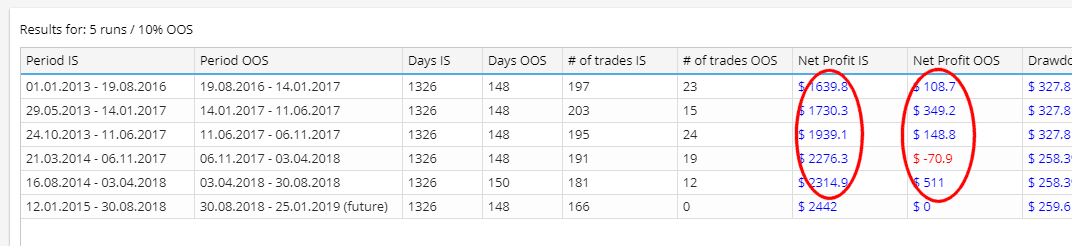# Description of advanced Walk-Forward values that can be used in filters / databank

Author: Mark Fric

January 18th, 2019

Rating:(2 votes, average: 5.00 out of 5)Loading...

There are some special stats computed during Walk-Forward optimization that you can use in filters or display in databank.

## Standard values computed for Walk-Forward optimization

These are all standard stats like Net profit, Number of trades, Sharpe ratio, etc. but computed from Walk-Forward optimization equity, not from main backtest.

You can get these values when you edit the column by double clicking on it and by choosing “Walk-Forward Matrix” or “Walk-Forward Optimization” in the From backtest selection.This means that the value will be taken from WF result.
In case of WF Matrix there are multiple WF Optimizations, so the value is taken from the first optimization.

### WF Results

These are standard values – for example resulting Net profit of the WF optimization.

### WF Stability

Stability values show performance in run vs in optimization part, they are always in %.

They are computed simply by making a sum of given values for IS and OOS part, normalizing it by the number of days (so that it is not dependent on length of each period), and computing its percentage of OOS versus IS result.

For example – Net Profit (WF Stability) tells us how much is Net profit performing in OOS (run) instead of IS (optimization) part.Value above 100% means that strategy performs better in run than in optimization part, value below 100% means that strategy performs worse in OOS (run) than in IS part (optimization) – this is expected, because we chose the best strategy in optimization part. You can expect WF Stability values to be below 100%.

Note that the values are normalized by dividing the resulting Net profit by number of days for its IS or OOS part, so it doesn’t matter how long is each period.

Usually we can expect that strategy performance on run part is worse than the optimized part, because optimized part was already optimized for the best performance.

The example of conditions below allow us to set boundaries how big performance decrease we are willing to accept:

• Net Profit (WF Stability) – Net profit performance in run vs in optimization part (in percent).
Value above 100% means that strategy performs better in run than in optimization part. Let’s say you specify condition WF Net Profit Stability > 60%. This means that performance in run part (after optimization) should be at least 60% of performance in optimization period.So, for example, if the strategy made \$1000 in optimization period, it should make at least \$600 or more after optimization period to pass this condition. This is important to evaluate because we want our strategy to perform well after we optimize it and this condition allows us to control this – in our condition we let the optimization pass only if the startegy performs at least at 60% of the optimized performance.
• Drawdown (WF Stability) – Drawdown in run vs optimization part (in percent).
Value above 100% means that strategy has worse drawdown in run than in optimization part. Let’s say you specify condition WF Drawdown Stability Stability < 200%.
This means that drawdown in run part (after optimization) should be less than 200% of drawdown in optimization period.So, for example, if the strategy had drawdown \$400 in optimization period, it should have drawdown smaller than \$800 after optimization period to pass this condition.This is an opposite example to Net Profit – we want drawdown to be as small as possible, but we can allow strategy to have worse drawdown after optimization.
• Return/DD (WF Stability) – Average Return/DD ratio in run vs optimization part (in percent).
Value above 100% means that strategy has better Return/DD ratio in run than in optimization part.
• Sharpe Ratio (WF Stability) – Average Sharpe ratio in run vs optimization part (in percent).
Value above 100% means that strategy has better Sharpe ratio in run than in optimization part.
• Profit Factor (WF Stability) – Average Profit Factor in run vs optimization part (in percent).
Value above 100% means that strategy has better Profit Factor in run than in optimization part.
• Annual % Return (WF Stability) – Annual % profit in run vs optimization part (in percent).
Value above 100% means that strategy performs better in run than in optimization part

### WF Score

WF Score is another special field that compares result of Walk-Forward optimization with result of original strategybacktest, and again returns percentage value of how much the optimization improved against original strategy.

For example, if you use Net Profit (WF Score), it compares Net profit of main backtest with Net profit of the optimization, and returns % improvement of optimization against original strategy.

If it returns 100% it means that final Net profit of Walk-Forward optimization is as same as Net profit of original strategy without optimization.Values over 100% mean that we achieved better results in Walk-Forward than in original strategy – it means that this strategy benefits from reoptimizations from time to time.

## Special values in Walk-Forward

There are several special values that are computed specifically for Walk-Forward results:

• Max Drawdown in one run – Maximal value of Drawdown in all the runs.
• Max % Drawdown in one run – Maximal % value of Drawdown in all the runs.
• Max profit in one run – Maximal value of Net Profit in all the runs.
• Max profit in one run as % of total – Maximal Net Profit in all the runs as percentage of total profit.
Ideally we don’t want any run to have too big % share on the final profit because it would mean the rest of optimiztion periods were not effective.
• Max Stagnation in % – Maximal stagnation (trades without new equity high) in days.
• Min trades in one run – the smallest number of trades in all the runs. Ideally we want each run to have reasonable number of trades to make the results valid.
• Percentage of profitable runs – How many Walk-Forward runs (in percent) were profitable

### Leave a Reply

Please Login to comment